# Common way to determine motor size/power in mechanical structures?

• Engineering
Homework Statement:
Find the minimum force for the motor
Relevant Equations:
(sum) Fx=0;
(sum) Fy=0;
(sum) Mo=0;
Hi all,

I have the following situation: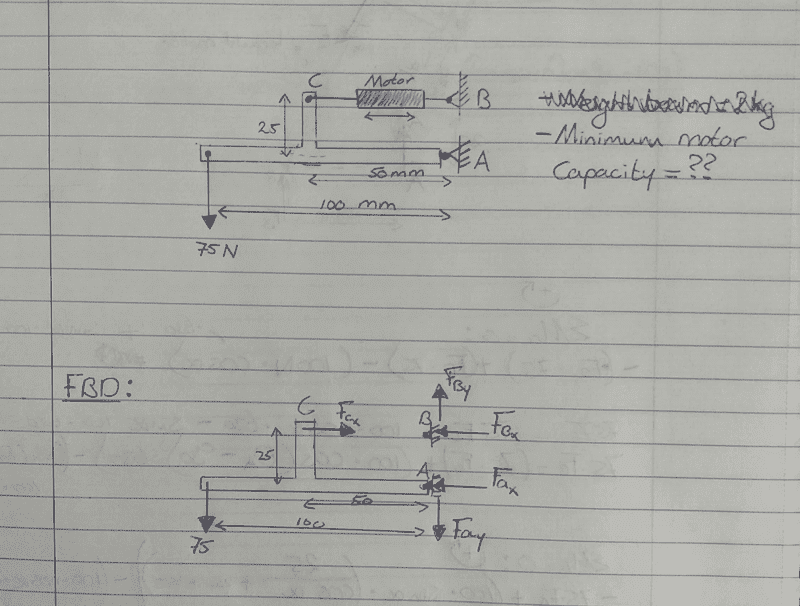I need to determine the minimum force needed for a motor to move the load (75 N) at the end of the beam. I added the forces in a FBD and did the sum of forces and moment calculations (see below). Is this calculation correct and what would be the next step for choosing the right motor? Searching through the internet I found a linear actuator (Linear actuator) with a 500 N capacity, is this the common way to handle a practical question ?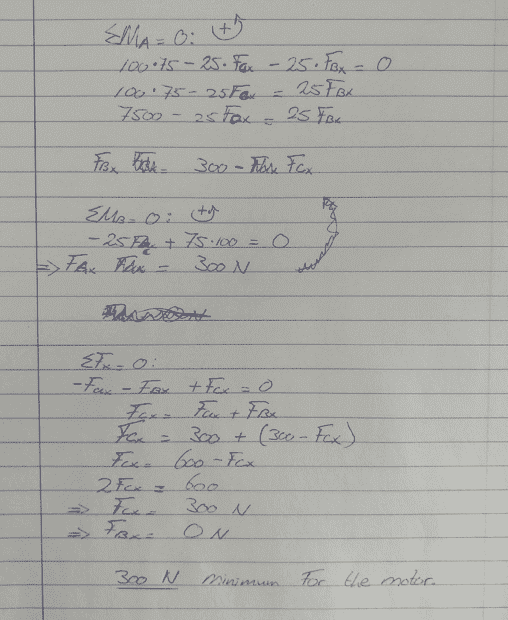Your question is about the motor force which is not the same thing as motor power. Since there is no information given about required speeds, nothing can be said about the required power.

If you want the 75 N to move up, all that is necessary is that the sum of moments bout the right end of the lever be positive taken clockwise.

If you want the 75 N load to move down, the reverse is true.

Ok,

I assume the speed applied on this system is 0.5 m/s. How can this be converted to the required power?

If you want the 75 N to move up, all that is necessary is that the sum of moments bout the right end of the lever be positive taken clockwise.
The direction of CW or CCW doesn't matter if the direction is applied on the same way through the calculation right? In the end a '-' sign will be given if the direction isn't applied on the right way.

Anyone who can help with this topic?

Thanks :)

jack action
Gold Member
Power = Force X Velocity. If you want the 75 N force to move at 0.5 m/s, then it requires 37.5 W of power. Since energy is conserved (so is power), then your actuator also needs to produce 37.5 W of power.

Don't forget to include friction at pivot points and the weight of your beam might also be a factor. If you need large accelerations, this may also be important. Analyze all possible situations, especially the extreme cases.

Power = Force X Velocity. If you want the 75 N force to move at 0.5 m/s, then it requires 37.5 W of power. Since energy is conserved (so is power), then your actuator also needs to produce 37.5 W of power.

Don't forget to include friction at pivot points and the weight of your beam might also be a factor. If you need large accelerations, this may also be important. Analyze all possible situations, especially the extreme cases.
In the first paragraph, Jack has said that energy and power are conserved. In the second paragraph, he says that it is important to include friction losses. These are contradictory statements.

His approach to calculating the required power is just fine except that, it is in that calculation that friction losses need to be included.

Power = Force X Velocity. If you want the 75 N force to move at 0.5 m/s, then it requires 37.5 W of power. Since energy is conserved (so is power), then your actuator also needs to produce 37.5 W of power.
Thanks. The location where the speeds applies doesn't play a role in this? To be clear what I mean, if I got a big truss construction included with a lot of beams with joints (so rotation is allowed), if I want this construction a speed of 5 m/s at the output, I can calculate the Power with only the force at the output multiplied by the speed?

I did a cross check with a linear actuator as an example. I found one on Amazon (Linear actuator) and they are referring to the "load capacity" which is capable for 330 lbs. Should this be converted to power? So this linear actuator can handle 330 lbs=1500 N. Makes:
1500 N x 0.5 m/s = 750 Watts. The actuator can produce 750 Watss of power for 0.5 m/s.

What do you mean with "Since energy is conserved"?

I re-calculated the FBD (see below). Now I find a force acting on the motor of 0 N ?? I am quite confused now.... The sketch includes a view of the Pivot joint which I have in mind, how should friction be added to this?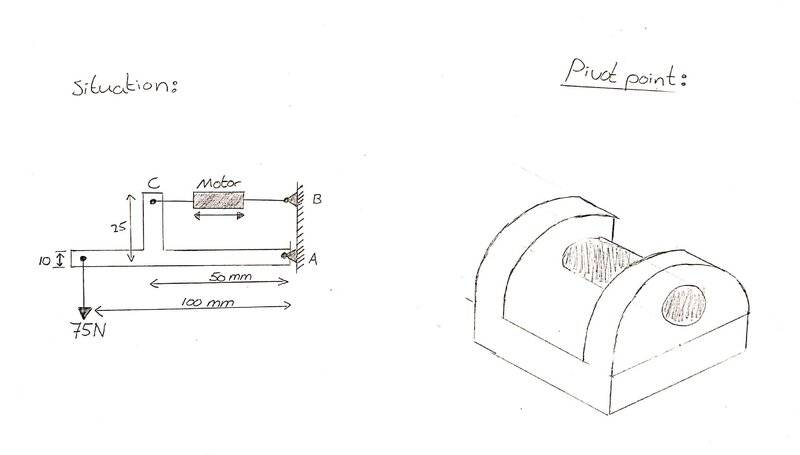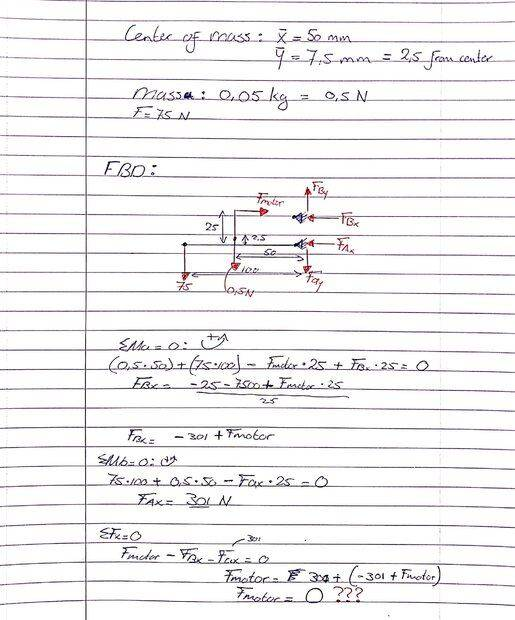jack action
Gold Member
To keep it simple, say you have only your 75 N force, moving at 5 m/s.

Doing a sum of moments about point A will give a horizontal force of 300 N at point C (basically, ##75\ N \times \frac{100\ mm}{25\ mm} = 300\ N##).

To find the horizontal velocity at point C , you first find the velocity at point C. The center of rotation is point A, thus the angular velocity is ##\frac{5000\ mm/s}{100\ mm} = 50\ rad/s##. Using the arm length AC - ##\sqrt{25^2 + 50^2} = 55.9017\ mm## - you can find that the velocity at point C is ##55.9017\ mm \times 50\ rad/s = 2795\ mm/s## (or 2.795 m/s). That velocity is perpendicular to the arm AC, i.e. at an angle of 26.5651° (##= atan\left(\frac{25\ mm}{50\ mm}\right)##). The horizontal component of that velocity is thus ##2.795\ m/s \times \sin 26.5651° = 1.25\ m/s##.

The power required at point C (horizontally only) is force times velocity, or 300 N X 1.25 m/s = 375 W. Surprise, surprise, it is the same as 75 N X 5 m/s = 375 W. That is because, in a system, energy cannot be destroyed, it is conserved. Since there is only one input point and one output point, they must be equal.

Now you can add other input forces, such as the frame inertia (frame mass times its acceleration, including gravity) or you can add a bearing friction moment at the different pivots. All of these will alter the horizontal force at point A. But the velocity will remain the same. If you calculated the sum of all these powers from each input point, it would also be equal to the new calculated linear actuator power.

The location where the speeds applies doesn't play a role in this?
No, it doesn't because of the mechanical advantage. When the force increases, the velocity decreases equally (and vice versa).
I found one on Amazon (Linear actuator) and they are referring to the "load capacity" which is capable for 330 lbs. Should this be converted to power? So this linear actuator can handle 330 lbs=1500 N. Makes:
1500 N x 0.5 m/s = 750 Watts. The actuator can produce 750 Watss of power for 0.5 m/s.
On the linked page, it also says that it moves at 5.7 mm/s. This means that its maximum power is 1468 N X 0.0057 m/s = 8.37 W. Not fast enough (< 1.25 m/s) and not powerful enough (< 375 W) for your application.
I re-calculated the FBD (see below). Now I find a force acting on the motor of 0 N ??
You shouldn't include forces at point B in your diagram. Only consider the forces acting on the frame.

•Mech_LS24
Thank you @jack action .

Why you're doing 5000 m/s / 100 mm? Is this the speed around point A? I looks to me more or less like a ratio? (I added my sketch below).

The power required at point C (horizontally only) is force times velocity, or 300 N X 1.25 m/s = 375 W. Surprise, surprise, it is the same as 75 N X 5 m/s = 375 W. That is because, in a system, energy cannot be destroyed, it is conserved. Since there is only one input point and one output point, they must be equal.
Thanks for this! This gives me a good understanding, substantiated with a calculation always helps me a lot :)

On the linked page, it also says that it moves at 5.7 mm/s. This means that its maximum power is 1468 N X 0.0057 m/s = 8.37 W. Not fast enough (< 1.25 m/s) and not powerful enough (< 375 W) for your application.
Make sense! I should have seen that.

You shouldn't include forces at point B in your diagram. Only consider the forces acting on the frame.
Maybe a stupid question but why should only forces acting on the frame be considered? The motor can kind of 'unload' his force into this pivot point B?

Now you can add other input forces, such as the frame inertia (frame mass times its acceleration, including gravity) or you can add a bearing friction moment at the different pivots. All of these will alter the horizontal force at point A. But the velocity will remain the same. If you calculated the sum of all these powers from each input point, it would also be equal to the new calculated linear actuator power.
Going to try this later this day. Really cool how things of mechanical engineering comes together!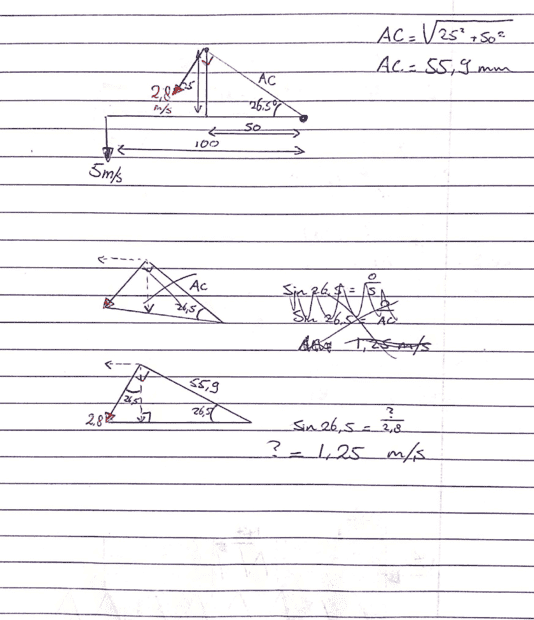jack action
Gold Member
Why you're doing 5000 m/s / 100 mm?
angular velocity = velocity / radius, or ##\omega = \frac{v}{r}##
Is this the speed around point A?
The angular speed to be precise.
I looks to me more or less like a ratio?
It is not a ratio because the units are different. It is a velocity divided by a length: ##\frac{5000\ \textbf{mm/s}}{100\ \textbf{mm}}##
Maybe a stupid question but why should only forces acting on the frame be considered? The motor can kind of 'unload' his force into this pivot point B?
Your system can be decomposed into 3 components: the frame, the actuator, and the ground. You should do a separate free body diagram for each of them to identify all forces.

angular velocity = velocity / radius, or
I think I got it. To check: Consider the following situation, see sketch. The red arrow around point A is the angular velocity. So if I take a closer look at the axle in point A and apply the 3.5 m/s, the axle at point A with rotate with an 'angular velocity' of 0.467 m/s?

Your system can be decomposed into 3 components: the frame, the actuator, and the ground. You should do a separate free body diagram for each of them to identify all forces.
I am sorry but I am a bit confused. What do you mean with 'ground'? I thought when I draw a FBD I should always consider every force apply to that particular structure/system? When I only consider the frame, my sketch in the beginning of this thread is wrong as well? Because the force from the motor (Fmotor) shouldn't be in the FBD?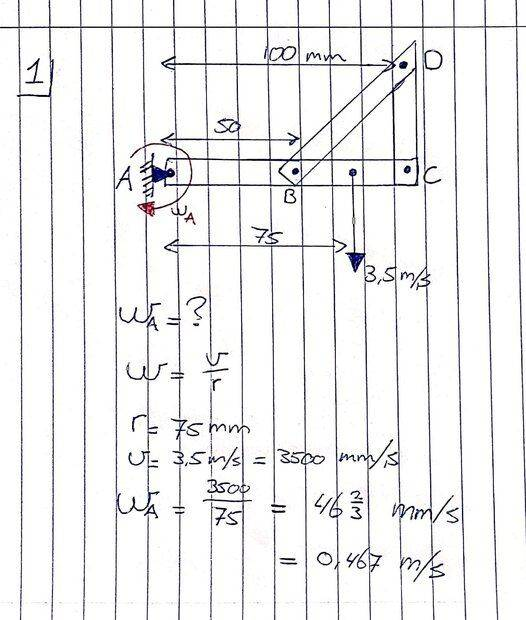jack action
Gold Member
So if I take a closer look at the axle in point A and apply the 3.5 m/s, the axle at point A with rotate with an 'angular velocity' of 0.467 m/s?
No. Units are important. You cannot mix mm and m. You must convert to the same unit:
$$\frac{3.5\ m/s}{0.075\ m} = 46.7\ rad/s$$
Or:
$$\frac{3500\ mm/s}{75\ mm} = 46.7\ rad/s$$
Note also that the angular velocity has the units of radian per second.

I thought when I draw a FBD I should always consider every force apply to that particular structure/system?
Just do a free body diagram for the frame (the force, and points A and C only) and another one for the actuator (points B and C only). Points A and B are also the reaction forces on the ground (the Earth), which we assume is stationary. Of course, once you have done the first two free body diagrams, the forces at points A and B will be equal and opposite on the ground.

You could also do one free body diagram for the frame/actuator assembly. But in this case, there are only the force, and points A and B present. Point C shouldn't be considered.

No. Units are important. You cannot mix mm and m. You must convert to the same unit:
Sorry, sloppy mistake from my side. So in this particular example I cannot calculate the velocity (m/s) at point A because point A is where the frame is rotating around?

But what makes it the difference between angular velocity and velocity? I found the following:
Velocity is the rate of change of displacement over time.

Whereas, angular velocity is the rate of change of angle with time.

e.g. If you are walking in a circle, Angular velocity is the rate at which you are turning, whereas velocity is the rate at which you are walking.
So I can imagine velocity is in a 'straight' line, while angular velocity has a 'rotating' point?

Just do a free body diagram for the frame (the force, and points A and C only) and another one for the actuator (points B and C only). Points A and B are also the reaction forces on the ground (the Earth), which we assume is stationary. Of course, once you have done the first two free body diagrams, the forces at points A and B will be equal and opposite on the ground.

You could also do one free body diagram for the frame/actuator assembly. But in this case, there are only the force, and points A and B present. Point C shouldn't be considered.
Going to try this later todayjack action
Gold Member
The SI unit of angular velocity is radian per second. A radian is a measure of angular displacement that corresponds to 57.3°.

Just like a car that has a velocity of 5 m/s will travel 5 meters for each second that passes, a wheel that has an angular velocity of 1 rad/s will revolve 57.3° for each second that passes.

Another unit for angular velocity that is more popular is the RPM which stands for Revolutions Per Minute. 1 revolution (360° or 6.28 rad) per minute (60 s) corresponds to 0.1047 rad/s.

The FBD's are shown below. I doubt about situation 3, I think as sketched is what you meant earlier?

Just like a car that has a velocity of 5 m/s will travel 5 meters for each second that passes, a wheel that has an angular velocity of 1 rad/s will revolve 57.3° for each second that passes.
Great example!

So if I take a closer look at the axle in point A and apply the 3.5 m/s, the axle at point A with rotate with an 'angular velocity' of 0.467 m/s?
So to correct this above, should be: The shaft/axle at point A will rotate with a angular velocity of 46.67 RAD/s or 445.75 RPM. The speed (v) at point A is 0 because length of the arm is 0.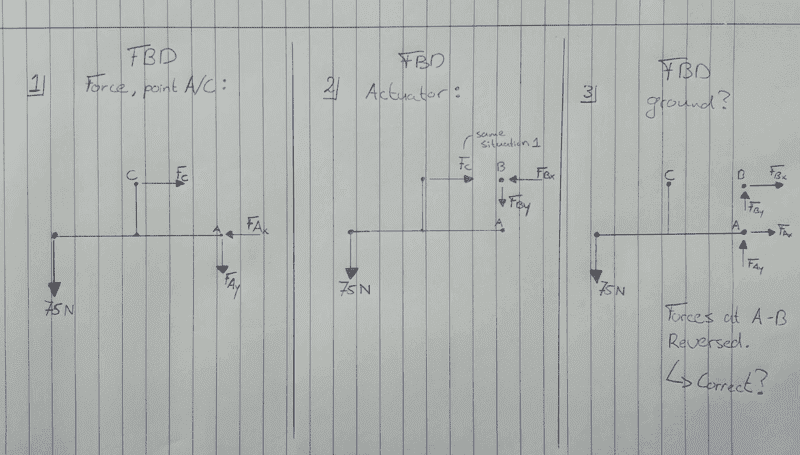jack action
Gold Member
The shaft/axle at point A will rotate with a angular velocity of 46.67 RAD/s or 445.75 RPM. The speed (v) at point A is 0 because length of the arm is 0.
Correct.
The FBD's are shown below. I doubt about situation 3, I think as sketched is what you meant earlier?
Sketch 1 is OK. (Usually ##F_{Ay}## should be upward, but that just means your value will end up being negative)

Sketch 2 should be a drawing of the actuator:
\begin{aligned} &C\ \ \ \ \ \ \ \ \ \ \ \ \ \ \ \ \ \ \ \ \ \ \ B \\ F_C \leftarrow\ \ \ &o------o\ \ \ \rightarrow F_B \end{aligned}
Note that ##F_C## of sketch 2 is equal and opposite to ##F_C## of sketch 1.

As for a free body diagram for the ground, forget about it, you don't need it. I just wanted you to understand that the ground plays a role as a link; A special link that is considered fixed.

•Mech_LS24
Thanks :)

Can I make it as the sketch below? So make one 'main' FBD with all forces occurs at the frame, then split it to the two FBD diagrams? I think the penny has been dropped if this is the case.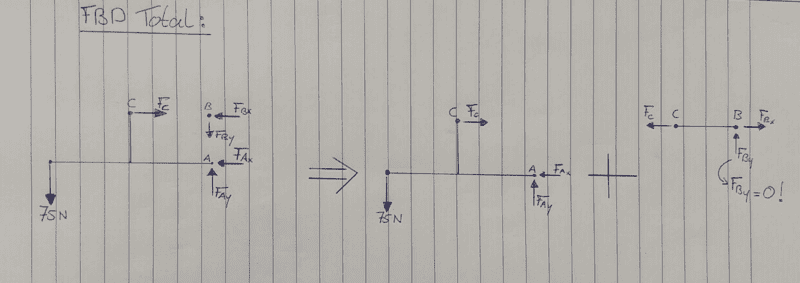jack action
Gold Member
On the left-hand side, you have to remove the force ##F_C## (which will be an internal force) and add the link ##CB##. It will still be a valid FBD for the whole assembly.

Hmm Okay, it should be like below? The actuator is replaced by a rigid connection?•jack action
@jack action Do you know a similar example of this? I think I do understand it know, but want to try to exercise a bit more with problems like this.

Pages 10 & 12 from this document;
This entire document should also be helpful.
Thanks :)

Besides, is it correct that the load doesn't affect the velocity? For example, I do increase the load from 75 N up to 150 N, when I re-calculated the velocity, it is still 1.25 m/s. On the other hand, the Power does increase while the load increases.

jack action